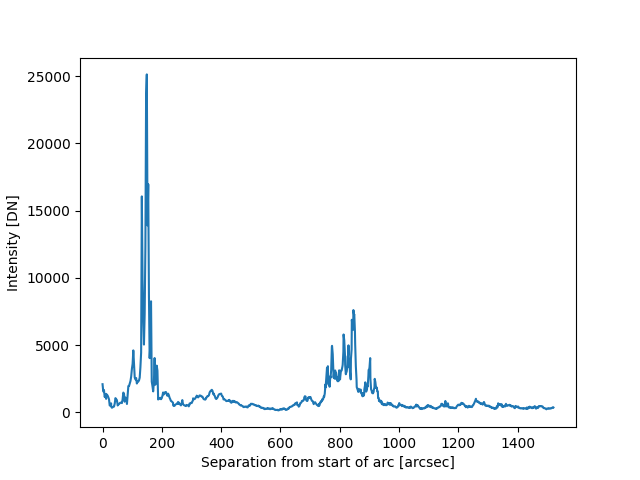# Drawing and using a Great Arc¶

How to define and draw a great arc on an image of the Sun, and to extract intensity values along that arc.

import matplotlib.pyplot as plt
import numpy as np

import astropy.units as u
from astropy.coordinates import SkyCoord

import sunpy.map
from sunpy.coordinates.utils import GreatArc
from sunpy.data.sample import AIA_171_IMAGE


m = sunpy.map.Map(AIA_171_IMAGE)


Let’s define the start and end coordinates of the arc.

start = SkyCoord(735 * u.arcsec, -471 * u.arcsec, frame=m.coordinate_frame)
end = SkyCoord(-100 * u.arcsec, 800 * u.arcsec, frame=m.coordinate_frame)


Create the great arc between the start and end points.

great_arc = GreatArc(start, end)


Plot the great arc on the Sun.

fig = plt.figure()
m.plot(axes=ax, clip_interval=(1, 99.99)*u.percent)
ax.plot_coord(great_arc.coordinates(), color='c')

plt.show()Now we can calculate the nearest integer pixels of the data that correspond to the location of arc.

pixels = np.asarray(np.rint(m.world_to_pixel(great_arc.coordinates())), dtype=int)
x = pixels[0, :]
y = pixels[1, :]


Get the intensity along the arc from the start to the end point.

intensity_along_arc = m.data[y, x]


Define the angular location of each pixel along the arc from the start point to the end.

angles = great_arc.inner_angles().to(u.deg)


Plot the intensity along the arc from the start to the end point.

plt.plot(angles, intensity_along_arc)
plt.xlabel(f'Distance along arc [{angles.unit}]')
plt.ylabel(f'Intensity [{m.unit}]')

plt.show()Total running time of the script: ( 0 minutes 1.129 seconds)

Gallery generated by Sphinx-Gallery